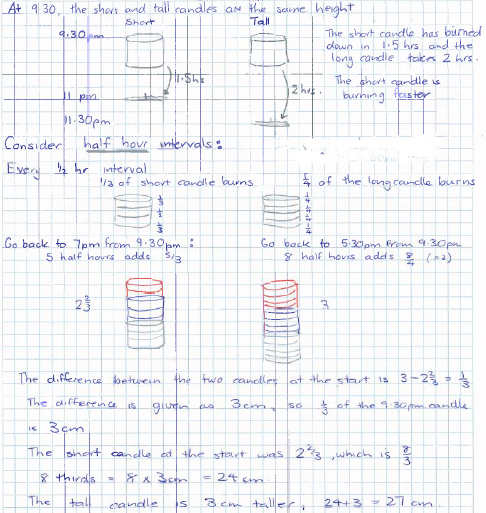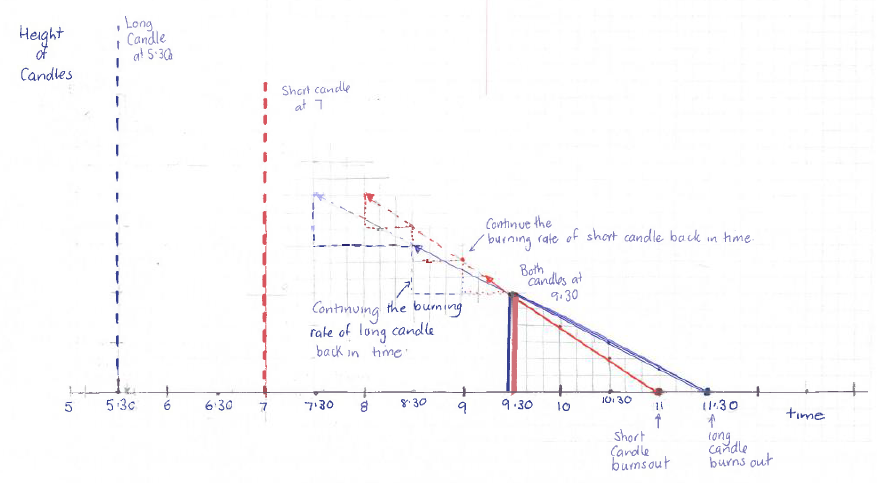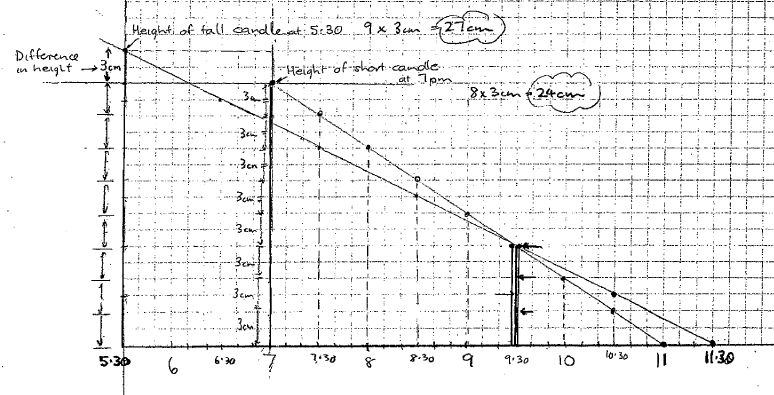#### You may also like### Golden Thoughts

Rectangle PQRS has X and Y on the edges. Triangles PQY, YRX and XSP have equal areas. Prove X and Y divide the sides of PQRS in the golden ratio.### At a Glance

The area of a regular pentagon looks about twice as a big as the pentangle star drawn within it. Is it?### Contact

A circular plate rolls in contact with the sides of a rectangular tray. How much of its circumference comes into contact with the sides of the tray when it rolls around one circuit?

# Burning Down

##### Age 14 to 16Challenge Level

There are lots of nice solutions to this problem, using a range of different methods:

Rhiannon sent in this very nice solution.

It takes the big candle $6$ hours to burn down, and it takes the smaller candle $4$ hours.

At $9 \colon 30$, the big candle has $\frac{1}{3}$ of its time left to burn, so is $\frac{1}{3}$ of the original length. The small candle has $\frac{3}{8}$ of its time left to burn, so is $\frac{3}{8}$ of its original length.

Since the candles are at the same height at this time, $\frac{1}{3}$ of the large candle is the same height as $\frac{3}{8}$ of the small candle.

Writing $l$ for the height of the large candle and $s$ for the height of the small candle, we get:$$\frac{1}{3}l=\frac{3}{8}s$$Therefore $l=\frac{9}{8}s$.

This means the difference in heights is $l-s = \frac{1}{8}s$. But we already know the difference in heights is $3\text{cm}$, so $\frac{1}{8}s=3\text{cm}$, or $s=24\text{cm}$. Therefore the small candle is $24\text{cm}$ tall, and this makes the large candle $27 \text{cm}$ tall.

This solution uses fractions and proportional reasoning to determine the heights of the candles:The next solution used a graphical method in order to find the height:It is also possible to use algebra, as in the final solution below:

Let the height of the shorter candle be $x$ and the height of the taller candle be $x + 3$. Assume that the short candle burns down at $a$ cm per hour and the tall candle burns down at $b$ cm per hour.

The candles are the same length at 9.30 so:$$x-\tfrac{5}{2}a=x+3-4b$$Also the short candle burns out after 4 hours so:$$4a=x$$and the tall candle burns out after 6 hours so:$$6b=x+3$$Using these three equations gives:$$x-\frac{\tfrac{5}{2}x}{4}=x+3-\frac{4(x+3)}{6}$$which, when solved, gives $x = 24\text{cm}$.

Finally, originally the shorter candle was 24cm and the longer one was 27cm.